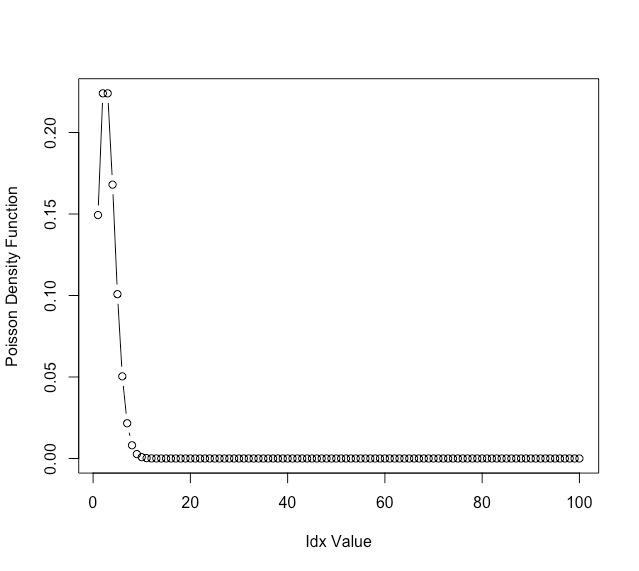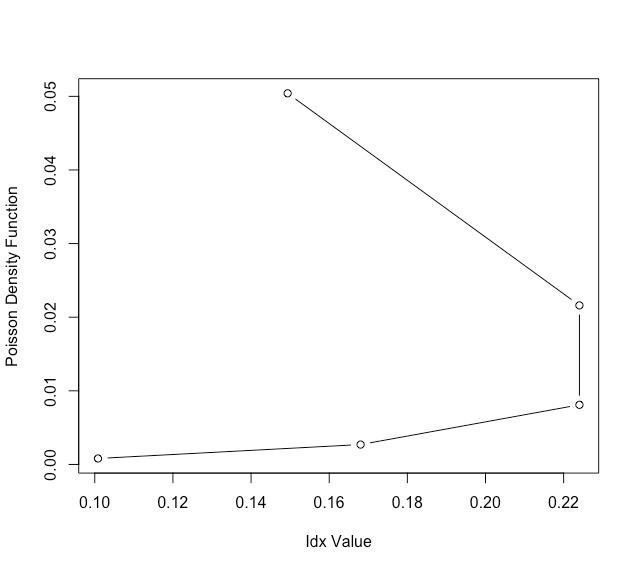GeeksforGeeks App
Open AppBrowser
Continue

# How to Draw Poisson Density Curve in R?

In this article, we are going to learn how to draw the Poisson density curve in the R programming language.

Function used:

dpois(): The dpois() method is used to accept the vector of quantiles and the vector of means as the input parameters. The dpois() method has the following syntax :

Syntax: dpois (q, m)

Arguments :

• q – Vector of quantiles.
• m – Vector of means.

## Computing Poisson density curve for vectors

To draw the Poisson density curve for vectors firstly we are declaring data points from 1 to 100 and specify the lambda value as 3 and after that  using the plot() method to plot the Poisson density curve on a graph with dpois() method inside it to accept the vector quantiles.

## R

 `# Declaring the data points``# from 1 to 100``data_points <-1:100``print``(data_points)` `# Specifying the lambda value``val <- 3` `# Plotting the data points``plot``(``dpois``(x= data_points,lambda= val),type=``"b"``,``     ``xlab = ``"Idx Value"``,``     ``ylab = ``"Poisson Density Function"``)`

Output:

> print(data_points)

   1   2   3   4   5   6   7   8   9  10  11  12  13  14  15  16  17  18  19  20  21  22  23  24

  25  26  27  28  29  30  31  32  33  34  35  36  37  38  39  40  41  42  43  44  45  46  47  48

  49  50  51  52  53  54  55  56  57  58  59  60  61  62  63  64  65  66  67  68  69  70  71  72

  73  74  75  76  77  78  79  80  81  82  83  84  85  86  87  88  89  90  91  92  93  94  95  96

  97  98  99 100## Computing the Poisson distribution function of the matrix

Here, we are creating the matrix of data points and using them to plot the Poisson density curve as same as above graph.

## R

 `# Declaring the data points``data_points <-``matrix``(1:20,ncol=4)``print``(data_points)``# Specifying the lambda value``val<- 3``# Plotting the data points``plot``(``dpois``(x= data_points,lambda= val),type=``"b"``,``     ``xlab = ``"Idx Value"``,``     ``ylab = ``"Poisson Density Function"``)`

Output:

> print(data_points)

[,1] [,2] [,3] [,4]

[1,]    1    6   11   16

[2,]    2    7   12   17

[3,]    3    8   13   18

[4,]    4    9   14   19

[5,]    5   10   15   20My Personal Notes arrow_drop_up